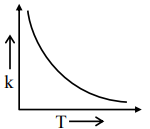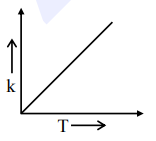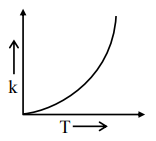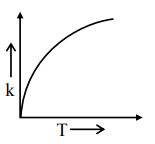# Which one of the following given graphs represents the variation of rate constantQuestion:

Which one of the following given graphs represents the variation of rate constant $(\mathrm{k})$ with temperature (T) for an endothermic reaction ?

1.2.3.4.Correct Option: , 3

Solution:

By observation we get this plot during measurable temperatures

Ans. $3^{\text {rd }}$ Option.